Next: Implementation Up: Second Cauchy theorem Previous: Second Cauchy theorem   Contents

Mathematical background

Let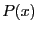be an univariate polynomial of degree: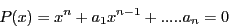with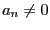. Let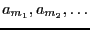with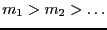the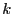strictly negative coefficients of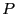. Then all the positive real roots ofverify :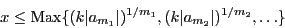Note that if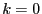all the roots are negative according to Descartes Lemma (see section 5.5.1).

Jean-Pierre Merlet 2012-12-20